Function Repository Resource:

# GaussianIntegerQ

Determine if a number is a Gaussian integer

Contributed by: Arnoud Buzing
 ResourceFunction["GaussianIntegerQ"][z] gives True is z is a Gaussian integer and False otherwise.

## Details and Options

A Gaussian integer is a number whose real and imaginary parts are both integers.

## Examples

### Basic Examples (4)

Zero is a Gaussian integer:

 In:=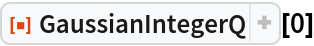Out=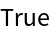Any integer is a Gaussian integer:

 In:=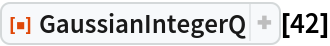Out=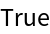A complex number with integer real and imaginary parts is a Gaussian integer:

 In:=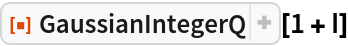Out=Non-integer numbers are not Gaussian integers:

 In:=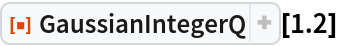Out=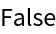In:=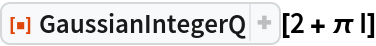Out=Arnoud Buzing

## Version History

• 1.0.0 – 29 April 2020